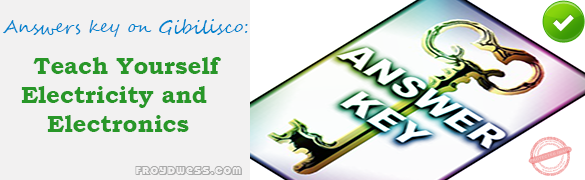# Resistors – Gibilisco MCQs in Electronics – Answers

(Last Updated On: December 8, 2017)Below are the answers key for the Multiple Choice Questions (MCQs) in Chapter 6: Resistors from the book Teach Yourself Electricity and Electronics, 5th edition by Stan Gibilisco.

1. Can be done using voltage dividers.

2. Current-limiting resistors.

3. Are connected across the capacitor in a power supply.

4. Are comparatively nonreactive.

5. In a high-power, direct-current circuit.

6. Has less reactance than a wirewound type.

7. A linear-taper potentiometer.

8. A logarithmic-taper potentiometer.

9. 5 dB.

10. 50 mW.

11. 100,000.

12. A rheostat can handle more current.

13. 7.4 percent.

14. 3,135 and 3,465 Ω.

15. 50.0 Ω.

16. 1/4 W.

17. Two 2.2 K Ω, 1-W resistors in parallel.

18. 2.2 K Ω.

19. 110 Ω.

20. 660 K Ω to 980 K Ω.

Rate this: